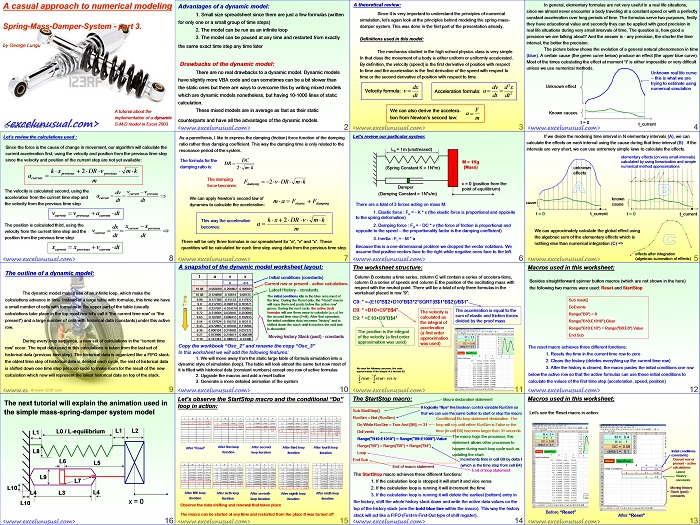# Casual Introduction to Numerical Methods – a spring-mass-damper system model – part#3

Here is the third part of a tutorial in both elementary dynamics and numerical methods. It is written at a basic level and it shows you how to set up a dynamic model for numerical solving of simple differential equations.   The dynamic model makes use of an infinite loop, which make the calculations advance in time. Instead of a large table with formulas, this time we have a small number of cells with formulas in the upper part of the table (usually calculations take place in the top most row let’s call it “the current time row” or “the present”) and a large number of cells with historical data (constants) under this active row.
During every loop sequence, a new set of calculations in the “current time row” occur. The input data used in this calculations is taken from the last set of historical data (previous time step). The historical data is organized like a FIFO stack: the oldest time step of historical data is deleted each cycle, the rest of historical data is shifted down one time step per loop cycle to make room for the result of the new calculation which now will represent the latest historical data on top of the stack. Download the model and hit Reset first and then RunStop. Enjoy, George[sociallocker][/sociallocker]

1.George Lungu says:
2.Vladan R. says: